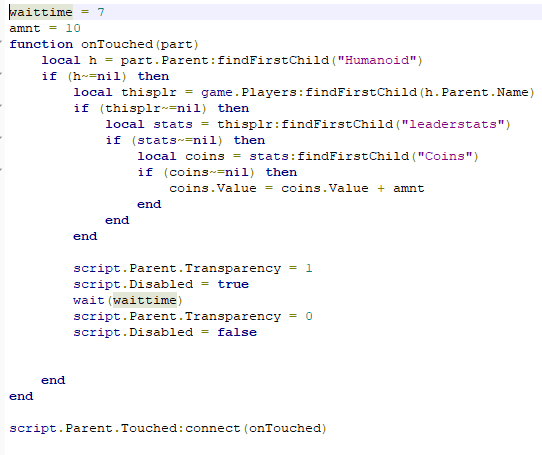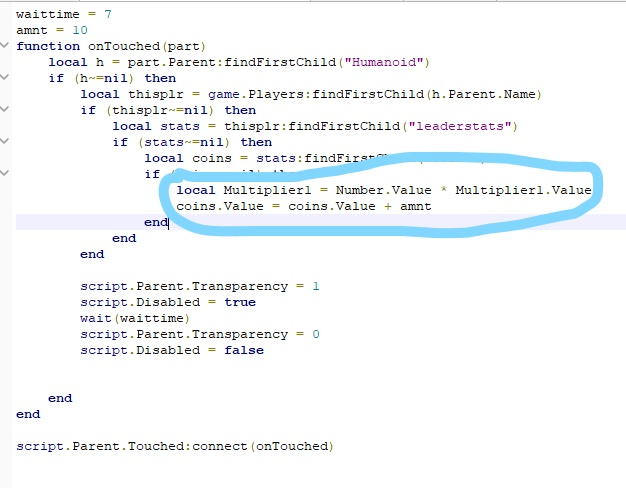# Help needed with pet multipliers (ASAP)

I’ve been working on a pet system, and I’m wondering how I would set up the pet multipliers to multiply the amount of coins you get every time you collect a coin. Here is the script I have for the coin.The multiplier is a NumberValue, called “Multiplier1”.
I already have everything else set up, except for this part. I am unsure of how to make it so that the Multiplier1 multiplies the coins that are earned i was thinking something like “coins.Value = coins.Value +amnt * Multiplier1.Value” but that didn’t work

Any help is appreciated!

4 Likes

I hope this makes sense to you:

``````local Multiplier = Number.Value * Multiplier.Value
``````
1 Like

Yep, I’ll try it out, that makes sense

1 Like

Sadly that didn’t work, i’m unsure why though

Can I see your script with it in it?

1 Like`````` coins.Value = coins.Value + (amnt * Multiplier1.Value)
``````
1 Like

ill try it out! thanks so much!

didn’t work, but theres nothing in the output

Throw a

``````print(Multiplier1.Value)
``````

Right before the

`````` coins.Value = coins.Value + (amnt * Multiplier1.Value)
``````

and tell me what it says.

(Multiplier1.Value)
coins.Value = coins.Value + (amnt * Multiplier1.Value)

like this?

No,

``````print(Multiplier1.Value)
coins.Value = coins.Value + (amnt * Multiplier1.Value)
``````

Workspace.Coin.Get Coins:12: attempt to index nil with ‘Value’

Oh ok, so do you have a pet system set up already?

If so, where do you specify what “Multiplier” the pet has.

You need to reference the Multiplier number for the specific pet inside your script.

thats what it said in the output

the pet system is already set up.
each pet has a folder named after them in replicated storage, which has a settings folder, which has the multiplier1 value.

in order starting at replicated storage, it would go. ReplicatedStorage - Pets - PetModels - Bat - Settings - Multiplier1

the Bat could be replaced with any other pet, and i need it to account for the combined value of all the equipped pets multipliers

Do you have a way set up to detect which pets a specific player has equiped?

yes, i have a way to detect it…